# Building a command line tool to generate Metroid passwords

By John Lekberg on October 17, 2020.

This week's blog post is about creating a command line tool that generates passwords for the 1987 game Metroid.

In Metroid, you are a bounty hunter that has been contracted to recover stolen bioweapons -- the "Metroids" -- from a group of aliens called the "Space Pirates".

Metroid was notable at the time for being one of the first games with a female protagonist.

The North American release of Metroid uses a password system instead of allowing you to save your progress to the cartridge. This was done because the ROM cartridges used by games for the Nintendo Entertainment System (NES) could not save game progress without using additional memory cards, which would increase the manufacturing cost.

Metroid's password system encodes the game state into a 144-bit password, represented as a 24-character string, which uses a custom 64-letter alphabet. The password system is documented in John David Ratliff's "Metroid Password Format Guide".

I created this tool so that I could generate custom passwords that allowed me to create various challenges for myself. E.g.

• Starting the game with all powers unlocked.
• Starting the game in a random location.
• Starting the game with some powers permanently locked and inaccessible.

In this week's post, you will learn:

• How to use set operations to combine different password features.
• How to also use set operations to check that data constraints are not violated.
• How to use bitwise arithmetic to encode the password.

# Script source code

``````#!/usr/bin/env python3

#
# Create passwords for the NES game Metroid (1987).
#
# Want to know what specific bits (e.g. 1, 64, 65, 66) mean?
# Refer to:
#
# Ratliff, John David. "Metroid Password Format Guide." 2012. emuWorks.

def MAIN():
import argparse

parser = argparse.ArgumentParser()
"--missiles",
type=int,
metavar="N",
help="number. 0 <= N <= 255",
)
"--location",
choices=pp_location.keys(),
help="Samus starts here",
)
args = parser.parse_args()

if args.missiles is not None:
if args.location is not None:

print(encoded[0:6], encoded[6:12])
print(encoded[12:18], encoded[18:24])

"""Represents a partially prepared password, with
certain bits being ON or OFF, and the rest of the bits
being undetermined.

PartialPassword objects can be combined as long as the
bit assignments don't clash (e.g. the same bit is both
ON and OFF).

The internal representation is a set of ON bits (public
attribute ".on") and a set of OFF bits (public attribute
".off").
"""

def __init__(self, *, on=(), off=()):
"""
Initialize a PartialPassword using supplied ON bits
and OFF bits.

on -- iterable of bit positions representing bits
set ON. (Default: no bits.)
off -- iterable of bit positions representing bits
set OFF. (Default: no bits.)

Verify that bits positions are between 0 and 127
(inclusive). Verify that no bit is both ON and OFF.
"""
s_on = frozenset(on)
s_off = frozenset(off)

raise ValueError(
f"Out of range (0-127) bits: {sorted(s_bad_range)}"
)

s_multi_state = s_on & s_off
if s_multi_state:
raise ValueError(
f"Multiple states for bits: {sorted(s_multi_state)}"
)

self.on = s_on
self.off = s_off

def combine(self, other):
"""Combine two PartialPassword object to create a
new PartialPassword object with all combined bits
set ON and OFF appropriately.
"""
raise TypeError(
)
on=self.on | other.on,
off=self.off | other.off,
)

def __or__(self, other):
return self.combine(other)

# Different starting locations.
pp_location = {
}

def pp_missiles(n):
"""
Create a PartialPassword object that gives Samus `n`
missiles.

n -- Number of missiles. 0 <= n <= 255.

The resulting PartialPassword object also sets the bit
for at least one missile tank ON. This is done because,
if no missiles tank bits are set ON, then the missile
counter is not shown in the game's user interface.
"""
if n not in range(0x100):
raise ValueError(
f"{n} missiles requested. Must be 0-255."
)

on = set()
off = set()
for i in range(8):
n_bit_i = ((0b1 << i) & n) >> i
missile_i = 80 + i
if n_bit_i == 1:
elif n_bit_i == 0:

# missile tank bit

# Represent the relationship between six-bit values and
#
# - To convert six-bit value -> character, use subscripts
#   (indexing).
# - To convert character -> six-bit value, use `str.index`.
metroid_alphabet = (
"0123456789"
"ABCDEFGHIJKLMNOPQRSTUVWXYZ"
"abcdefghijklmnopqrstuvwxyz"
"?-"
)

def metroid_encode(partial_pwd):
"""Convert a PartialPassword object into a 24-character
str object that can be read and typed into Metroid's

Pseudocode:

-> 16 byte integer
-> bytes object (little endian)
- Calculate shift byte and checksum byte.
- Shift byte is always 0 to simplify encoding.
- Reversed [data, shift, checksum]
-> bytes object
-> 18 byte integer (little endian)
-> 24-character string.
"""
data = 0
for i in partial_pwd.on:
data |= 1 << i
data_B = data.to_bytes(16, "little")

shift = 0
checksum = sum(data_B) & 0xFF

pwd_B = bytes(reversed([*data_B, shift, checksum]))
pwd = int.from_bytes(pwd_B, "little")

pwd_alpha = ""
for i in reversed(range(0, 144, 6)):
j = ((0b111_111 << i) & pwd) >> i
pwd_alpha += metroid_alphabet[j]
return pwd_alpha

if __name__ == "__main__":
MAIN()
``````
``````\$ metroid-password --help
``````
``````usage: metroid-password [-h] [--missiles N]
[--location {brinstar,norfair,
kraid_lair,ridley_lair,tourian}]

optional arguments:

-h, --help            show this help message and exit
--missiles N          number. 0 <= N <= 255
--location {brinstar,norfair,kraid_lair,ridley_lair,tourian}
Samus starts here
``````

# Using the script to generate passwords

• I want to start in Brinstar with 202 missiles:

``````\$ metroid-password --location brinstar --missiles 202
``````
``````0W0000 000000
0Ce000 00003C
``````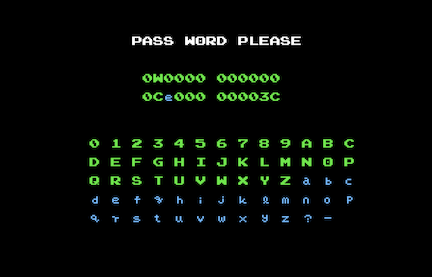• I want to start in Norfair with 250 missiles:

``````\$ metroid-password --location norfair --missiles 250
``````
``````0W0000 000001
0Fe000 00003z
``````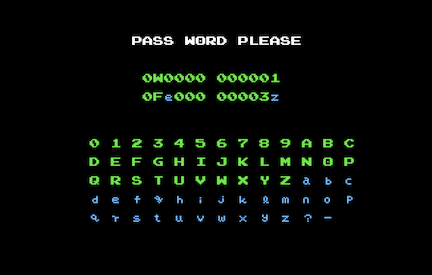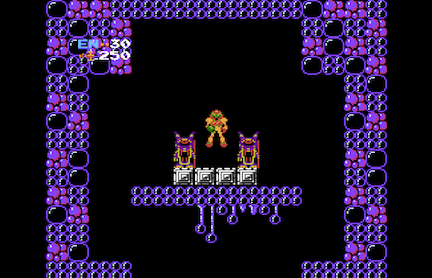• I want to start in Kraid's Lair with 160 missiles:

``````\$ metroid-password --location kraid_lair --missiles 160
``````
``````0W0000 000002
0A0000 00002a
``````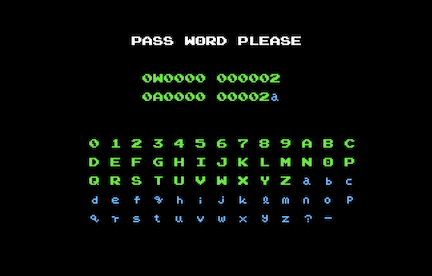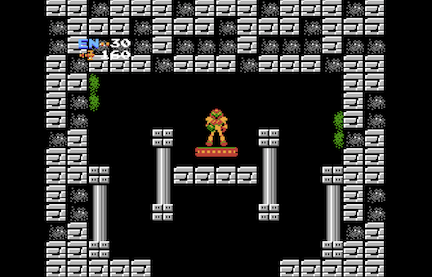• I want to start in Ridley's Lair with 100 missiles:

``````\$ metroid-password --location ridley_lair --missiles 100
``````
``````0W0000 000004
06G000 00001g
``````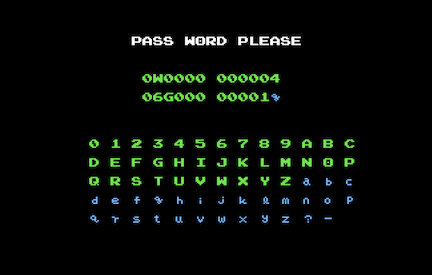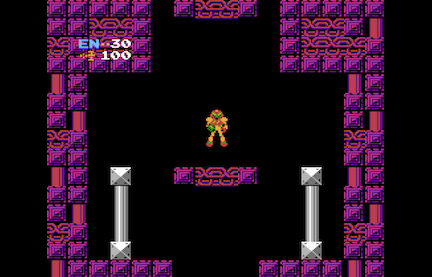• I want to start in Tourian with 76 missiles:

``````\$ metroid-password --location tourian --missiles 76
``````
``````0W0000 000003
04m000 00001H
``````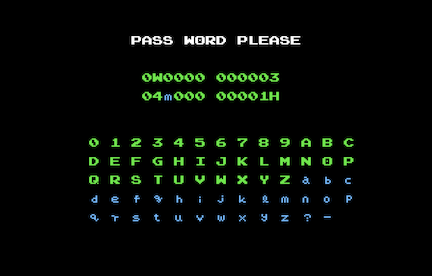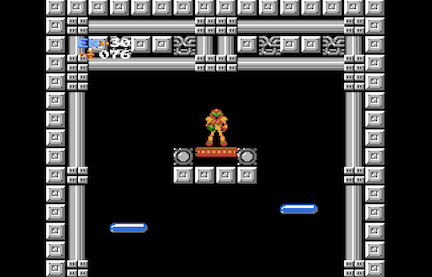# How the script works

I use argparse to parse the command line arguments.

A `PartialPassword` object represents a partially prepared password, with certain bits being ON or OFF, and the rest of the bits being undetermined:

• I use frozensets because they are immutable and because set operations simplify the code in this class.

• I use set.difference and the built-in function range to check if any bit positions are out of range. (The valid positions for a 16-byte number are 0 to 127.)

• I use set.intersection (the `&` operator) to check if any bits are both ON and OFF.

• I use set.union (the `|` operator) to combine ON bits and OFF bits in the `combine` method.

• I implemented the special method __or__ (which calls the `combine` method) because this gives me access to the `|` operator and allows me to write

``````x |= y
``````

``````x = x.combine(y)
``````

`pp_location` is a dictionary that maps location names (str objects) to `PartialPassword` objects. I determined the ON and OFF bits by referencing the Metroid Password Guide. (See section 4.5, "Known Password Bits".)

`pp_missiles` creates a `PartialPassword` object that represents the number of available missiles as an 8-bit byte at bit positions 80 to 87: (See Metroid Password Guide section 4.5, "Known Password Bits".)

• `0x` is Python's syntax hexadecimal numeric literals. E.g.

``````0xF3
``````
``````243
``````
• `0b` is Python's syntax for binary numeric literals. E.g.

``````0b1101
``````
``````13
``````
• The formula `((0b1 << i) & n) >> i` extracts 1 bit from the int `n` at position `i`. (See also `metroid_encode`, which uses a variation of this formula to extract 6 bits instead of 1 bit.)

• I set a missile tank bit ON because the game's user interface (UI) will not display a missile counter unless at least one missile tank is taken.

`metroid_alphabet` is a string that represents the 64-character alphabet used by Metroid's password system:

• I got this information from the Metroid Password Guide (section 4.2, "The Metroid Alphabet").
• I can convert a six-bit integer into a character using subscription (e.g. `metroid_alphabet[0b110101]`).
• I can convert a character into a six-bit integer using str.index.

`metroid_encode` encodes a `PartialPassword` object into a 24-character str ready to be entered into Metroid's password system:

• I convert between bytes objects and int objects using int.to_bytes and int.from_bytes using little-endian (LE) byte order.

• To lay out the data (16 B), shift (1 B), and checksum (1 B) into the password (18 B), I use iterable unpacking (the `*` operator). E.g.

``````x = [1, 2, 3]
[4, x, 5, x]
``````
``````[4, [1, 2, 3], 5, [1, 2, 3]]
``````
``````[4, *x, 5, *x]
``````
``````[4, 1, 2, 3, 5, 1, 2, 3]
``````

I also use the built-in function reversed to reverse the layout into the correct order.

• I use `reversed(range(0, 144, 6))` in the loop that creates `pwd_alpha` from `pwd`. I need to loop over this range of numbers in reversed order, and I prefer using `reversed(range(...))` because just using `range` would result in this expression: `range(138, -6, -6)`. For me, it's easier to read `reversed(range(0, 144, 6))` than `range(138, -6, -6)`.

• The formula `((0b111_111 << i) & pwd) >> i` extracts 6 bits from the int `pwd` at position `i`. (See also `pp_missiles`, which uses a variation of this formula to extract 1 bit instead of 6 bits.)

• This uses the binary bitwise operations `<<` and `&`.

• The underscore `_` is a digit separator. E.g.

``````860_834_212
``````
``````860834212
``````
``````860_834_212 == 860834212
``````
``````True
``````

(See numeric literals for details.)

# In conclusion...

In this week's post you learned how to create a password generator for Metroid. You learned how to use set operations to combine partial passwords and check that constraints were not violated. And you used bitwise arithmetic to encode the passwords.

My challenge to you:

In Metroid, you can collect power ups like the "Ice Beam" or "Bombs".

Add more `PartialPassword` objects for allowing Samus to start the game with these power ups: Bombs, High Jump Boots, Long Beam, Screw Attack, Maru Mari, Varia Suit, Wave Beam, and Ice Beam. (See the Metroid Password Guide, section 4.5, "Known Password Bits", for details.)

If you enjoyed this week's post, share it with your friends and stay tuned for next week's post. See you then!

(If you spot any errors or typos on this post, contact me via my contact page.)